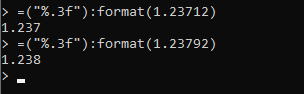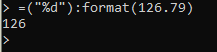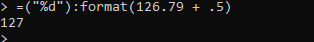# Rounding numbers on GUIs to the nearest hundredth

1. What do you want to achieve? I want to have my decimal rounded to the nearest 2nd decimal point.

2. What is the issue?

``````player.PlayerGui.Stats.Frame.Upgrades.UpgradeSpeed.Text = player.Stats.Speed.Value /70
``````

this script can sometimes output some number with a massive amount of decimals and I can’t think of a way to solve this.

1. What solutions have you tried so far? I tried looking for solutions on the devforum but all I could find was for rounding to the nearest whole number and shortening numbers (example 2k)
4 Likes

I believe what you could do here is divide your number by 100, as to make it a 2 point decimal, use either your math.floor() or math.ceil() rounding option and then times it by 100 again. This way, you can round it as a decimal and then get your original number back.

I tested it in studio and it seemed to work, so your code would look something like this.

``````player.PlayerGui.Stats.Frame.Upgrades.UpgradeSpeed.Text = math.floor((player.Stats.Speed.Value / 100)) * 100
``````

Edit: Sorry, misread your question, I’ll reconfigure this code to make it to 2 d.p

Edit 2: After some googling, I found this formula, which will return your number to 2 d.p.
`math.floor( (val * 10^num) + 0.5) / (10^num))`

I hope it works for you!4 Likes
``````script.Parent.Text = "+" .. Damage.Value /70 .. " speed for " .. Damage.Value *300 .. " Coins."
``````

the “val” and “num” seem to be variables but what do I fill in for them, is “val” the “damage.value” and num the “70” I tried

``````"+" .. math.floor( (Damage.Value * 10^70) + 0.5) / (10^100)
``````

but that outputted “+1e-30”, a number I have never seen in my life

That’s because you’re doing 10 to the power of 70 and dividing it by 10 to the power of 100.

num is simply how many d.p you want, so num would equal 2 in this situation. Sorry for the confusion.

1 Like

For anyone confused how `math.floor(x * 10^n) / (10^n)` actually works here is an explanation.

The `x * 10^n` is meant to shift `x` by `n` places. So if you have the number `10.25` and multiply by `10^2` which is `100` that leaves you with `1025`. Given this logic you could now floor the number which will cut off the remaining decimal portion if there was any and then divide by the original `10^n` because you want to put your number back to the same significant figures.

This works if you still need it.

``````local roundDecimals = function(num, places) --num is your number or value and places is number of decimal places, in your case you would need 2

places = math.pow(10, places or 0)
num = num * places

if num >= 0 then
num = math.floor(num + 0.5)
else
num = math.ceil(num - 0.5)
end

return num / places

end

print(roundDecimals(142.165, 2)) --> 142.17
``````
13 Likes

this problem is very simple to solve!

``````local Number = 0  -- put your number here
TextLabel.Text = string.format('%.2f%% of Speed', Number)
``````

I hope I have been helpful.in the place of ("%.2f%%), you can change the value “2” to how many decimal places you want to appear

2 Likes

He wanted to round the decimals though

the `%f` pattern automatically does decimal rounding.1 Like
``````math.floor( (Speed.Value * 10^2) + 0.5) / (10^2)
``````

IDK whats wrong with my script! the speed value is 16 and this outputs 16! It is supposed to output less then one

Edit: this now has a solution! thanks everyone!

Doesn’t seem to round them properly all the time from what I’ve tested though, I tried printing out the same number (14.4215) using both methods and got out 14.421 with this method above and 14.422 with the one I posted. But I guess that doesn’t really matter.

``````local percentage = Speed.Value / 70 * 100

print((math.floor(percentage * 100 + 0.5) / 100).."%")
-- OR
print(("%.2f%%"):format(percentage))
``````

The string formatting method will keep the “.00” even if it’s a round number, the math.floor method doesn’t.

1 Like

Cheat code:
Use the `%d` pattern instead to remove the padding!It does not do rounding though, it just removes the decimal portion. To do rounding add `.5` to `x`;

`("%d"):format(x + .5)`(For anyone wondering this works because if the first decimal place is greater than .5 that means you round up, and adding .5 effectively rounds it up and on the floor portion of the math (%d pattern) the decimal portion gets removed)